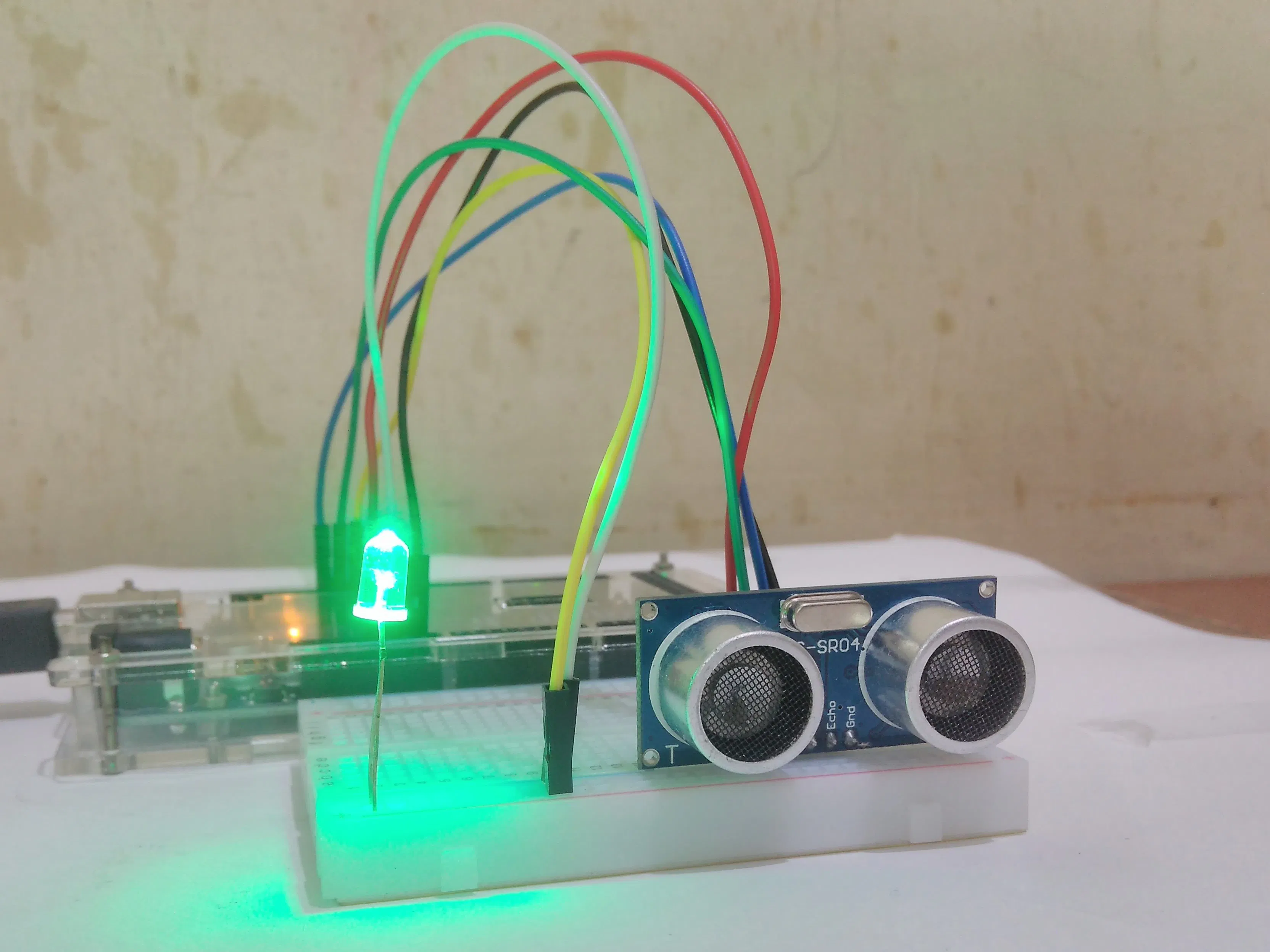# Ground Proximity Sensing with Ultrasonic Sensor

It is a miniature counterpart of radio altimeter used on commercial aircrafts to be used with RC aircrafts and multirotors.

BeginnerFull instructions provided8,967## Things used in this project

### Hardware componentsArduino UNO & Genuino UNO
×1Ultrasonic Sensor - HC-SR04 (Generic)
×1LED (generic)
×1

## Schematics

### circuitDiagram

The fritzing drawing of circuit diagram

## Code

Arduino
A code to sense rate of change of altitude of aircraft above ground in form of blinking of LED
```int triggerpin=10;
int echopin=11;
long duration;
long distance;
long x;
long x1=45;
int z;
void setup() {
// put your setup code here, to run once:
pinMode(triggerpin,OUTPUT);
pinMode(echopin,INPUT);
pinMode(9,OUTPUT);
Serial.begin(9600);
}

void loop() {
// put your main code here, to run repeatedly:
digitalWrite(triggerpin,LOW);
delayMicroseconds(2);
digitalWrite(triggerpin,HIGH);
delayMicroseconds(10);
digitalWrite(triggerpin,HIGH);
duration=pulseIn(echopin,HIGH);
x=(duration/2)/29.1;
Serial.print("Distance :");
Serial.println(x);
z=(x1-x)/2;
x1=x;
Serial.print("\t z :");
Serial.println(z);

if(z<-10)
{
analogWrite(9,25);
delay(1000);
analogWrite(9,LOW);
delay(1000);
}
else if(z>6)
{
analogWrite(9,25);
delay(10);
analogWrite(9,LOW);
delay(10);
}
else
{
z=map(z,-10,6,1000,5);
analogWrite(9,25);
delay(z);
analogWrite(9,LOW);
delay(z);
}
}
```

### stable_ultrasonic_brightness

Arduino
To measure the height and change brightness of LED accordingly.
```int triggerpin=10;
int echopin=11;
long duration;
long distance;
long x;
void setup() {
// put your setup code here, to run once:
pinMode(triggerpin,OUTPUT);
pinMode(echopin,INPUT);
pinMode(9,OUTPUT);
Serial.begin(9600);
}

void loop() {
// put your main code here, to run repeatedly:
digitalWrite(triggerpin,LOW);
delayMicroseconds(2);
digitalWrite(triggerpin,HIGH);
delayMicroseconds(10);
digitalWrite(triggerpin,HIGH);
duration=pulseIn(echopin,HIGH);
x=(duration/2)/29.1;                //1/29.1=0.034 ~ speed of sound converted into centimeters per microsecond
Serial.print("Distance :");
Serial.print(x);
x=map(x,0,100,255,0);      //mapping the intensity as per distance
Serial.print("\t");
Serial.print(x);
Serial.println();
if(x<255 && x>10)                 //code stability condition
{
analogWrite(9,x);
}
else if (x<10)
{
analogWrite(9,0);
}
else
{
analogWrite(9,0);
}
}
```